# Squeeze Theorem Example

Previous: Squeeze Theorem for Sequences

Next: Example: {rn}

## Example

Determine whether the sequence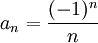converges.

## Complete Solution

Sinceand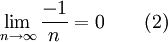andwe obtainby the Squeeze Theorem for infinite sequences. Therefore, the given sequence converges.

## Explanation of Each Step

### Steps (1) and (2)

To apply the squeeze theorem, we need two functions. One function must be greater than or equal tofor all n, so we can use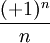This sequences has the property that its limit is zero.

The other function that we must choose must be less than to or equal to an for all n, so we can use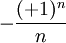This sequence also has the property that its limit is zero.

There is nothing special about the number zero. We need two sequences that have the same limit at infinity, which in this case happens to be zero.

### Step (3)

Our two sequences have the necessary property that one is greater than or equal to an for all n, and the other is less than or equal to an for all n.

### Step (4)

Our chosen sequences meet the requirements for the Squeeze Theorem to be applied.

## Possible Challenge Areas

### Knowing When to Use the Squeeze Theorem

We often use the Squeeze Theorem whenever we can easily create two sequences that bound the given sequence and have the same limit. In this example, the functionsandsatisfy these conditions.

### Knowing What Sequences to Choose

To apply the squeeze theorem, one needs to create two sequences. Often, one can take the absolute value of the given sequence to create one sequence, and the other will be the negative of the first.

For example, if we were given the sequence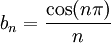we could choose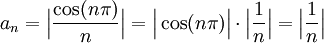as one sequence, and choose cn= - an as the other. These sequences would satisfy the conditions we need to apply the theorem (they both tend to the same limit as n tends to infinity).

Previous: Squeeze Theorem for Sequences

Next: Example: {rn}